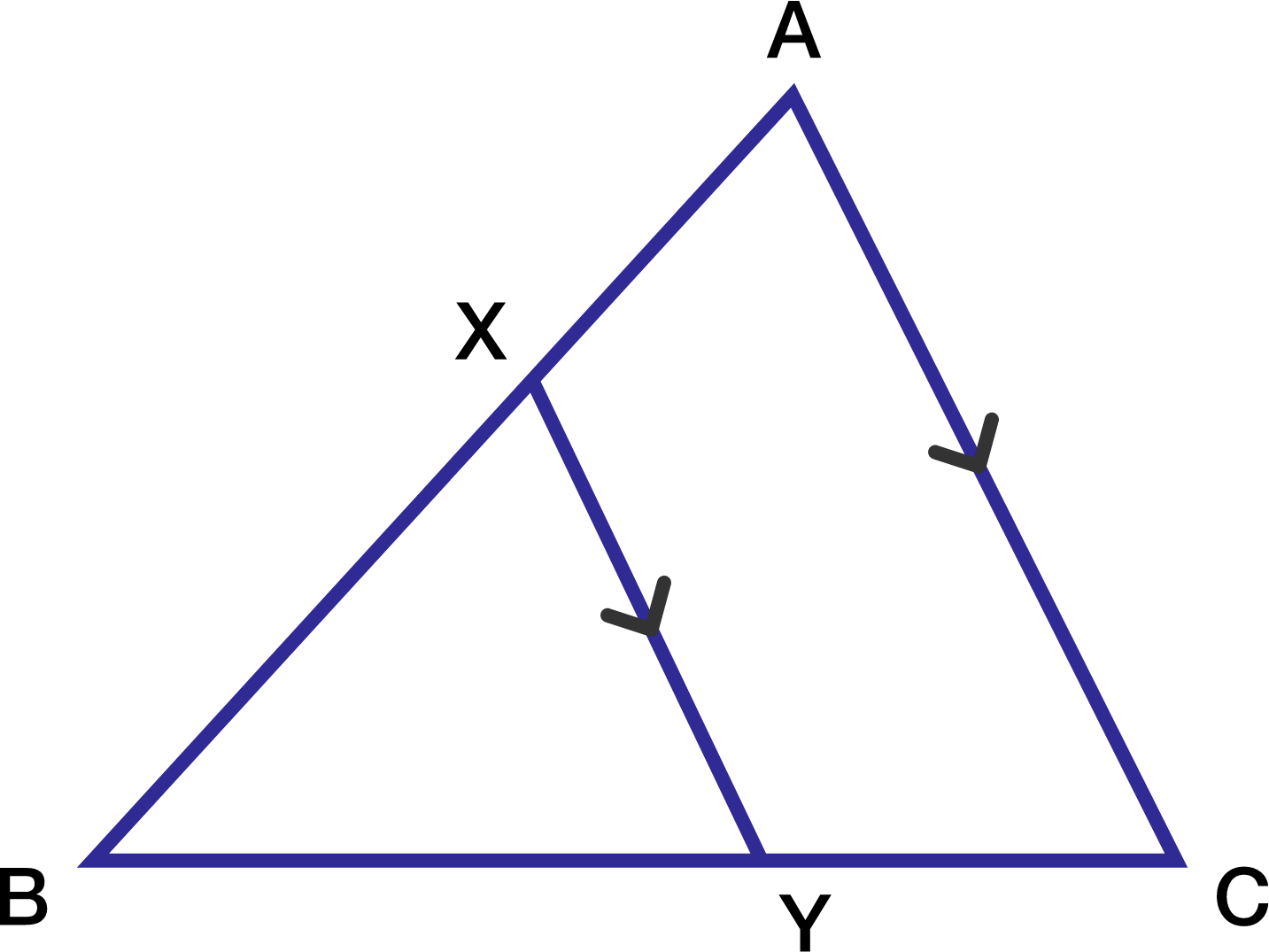# A cool geometry problem!

Geometry Level 2In the adjoining figure, $XY$ is parallel to $AC$. If $XY$ divides the triangle into two halves with equal area, compute $\dfrac{AX}{AB}$.

×

Problem Loading...

Note Loading...

Set Loading...